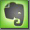BTU - British Thermal Unit

British Thermal Unit
The basic unit of heat energy. One Btu is the amount of heat needed to heat one pound of water one degree Fahrenheit at one atmosphere pressure, equivalent to 251.997 calories. One million Btu (1 MBtu) is about the energy equivalent of one person's labor in one year.

One kWh (kilowatthour) = 3412 BTU

 1 kilowatt-hour (kWh) is equivalent to 3412 British Thermal Units (BTU) 1000 watt (W) = 1 kilowatt (kW) is equivalent to 3412 British Thermal Units per hour (BTU/h) 1000 British Thermal Units (BTU) is equivalent to 0,293 kilowatt-hour (kWh)

The heat dissipation of an electrical device is equal to the AC power dissipation per unit of time. Technical specs often state how many BTU are generated per hour by the individual device.
All electrical power consumed by a device is finally converted to heat at 100% efficiency, may be not at the device itself because the device can transport power to another device. But always finally electrical watts consumed equal heat watts produced. A power supply using 50 watts finally produces 50 watts of heat.

100 watts electrical power equals 100J of energy per second. Watt is the unit of power. Joule is the unit of energy. Power is not energy ....

Heat dissipation in BTU/sec is calculated: by multiplying the AC-power (in kW) dissipation by 3.41214, divided by 3.6

Heat dissipation in BTU/hour is calculated: by multiplying the AC-power (in W) dissipation by 3.41214

Example:
10 kW => 10 kW * 3.41214 / 3.6 = 9.48 BTU/sec
10 kW => 10.000 W * 3.41214 = 34,121.4 BTU/hour

See the BTU calculator of TranslatorsCafe.com